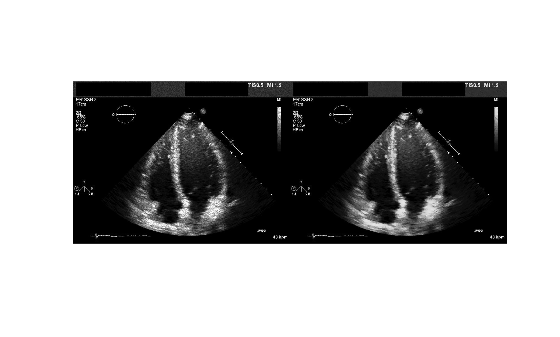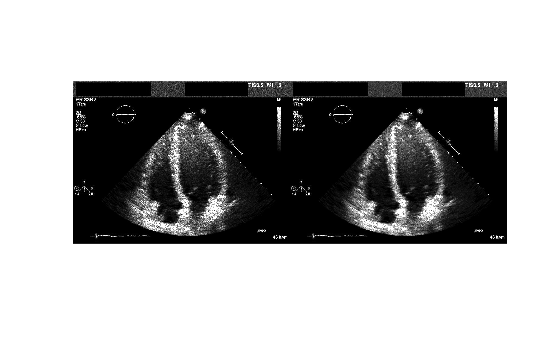# specklefilt

Filter image using speckle-reducing anisotropic diffusion

Since R2022b

## Syntax

``J = specklefilt(I)``
``J = specklefilt(I,Name=Value)``

## Description

Coherent imaging modalities, such as ultrasound images, are prone to degradation because of the interference of the transmitted waveform and its echoes. This degradation is called speckle, and is a form of multiplicative noise. The `specklefilt` function uses a speckle-reducing anisotropic diffusion (SRAD) algorithm to reduce the speckle in an image.

example

````J = specklefilt(I)` filters the image `I` using SRAD and returns the filtered image `J`.```

example

````J = specklefilt(I,Name=Value)` fine-tunes the behavior of the SRAD algorithm using one or more optional name-value arguments.```

## Examples

collapse all

Import an ultrasound image into the workspace.

`I = imread("heartUltrasoundImage.png"); `

Filter the image using the speckle-reducing anisotropic diffusion algorithm with default parameters.

`J1 = specklefilt(I);`

You can increase the smoothing the filter performs on the image by increasing the degree of smoothing of the algorithm. Specify a degree of smoothing of 0.6, increased from the default value of 0.2.

`J2 = specklefilt(I,DegreeOfSmoothing=0.6);`

You can also increase the smoothing by increasing the number of iterations performed by the algorithm. Specify for the algorithm to perform 50 iterations, increased from the default value of 30.

`J3 = specklefilt(I,NumIterations=50);`

Filter the image using the SRAD algorithm with both an increased degree of smoothing and greater number of iterations. Set the degree of smoothing to 0.6 and the number of iterations to 50.

`J4 = specklefilt(I,DegreeOfSmoothing=0.6,NumIterations=50);`

Display the original image alongside the denoised images, and inspect the different smoothing levels.

```figure montage({I J1 J2 J3 J4})```Import an ultrasound image into the workspace.

`I = imread("heartUltrasoundImage.png"); `

Corrupt the image with multiplicative speckle drawn from a uniform distribution.

`Inoise = imnoise(I,"speckle");`

Filter the corrupted image to remove speckle. Display the corrupted and denoised images.

```J = specklefilt(Inoise,DegreeOfSmoothing=0.6,NumIterations=50); figure montage({Inoise J})```Compute the structural similarity of the denoised image `J` with the original image `I`.

`disp(ssim(I,J))`
``` 0.9330 ```

Corrupt the image with multiplicative speckle drawn from a Rayleigh distribution.

```I = im2double(I); Inoise = I.*raylrnd(1,size(I));```

Filter the corrupted image to remove speckle. Display the corrupted and denoised images.

```J = specklefilt(Inoise,DegreeOfSmoothing=0.6,NumIterations=50); figure montage({Inoise J})```Compute the structural similarity between the denoised image `J` and the original image `I`.

`disp(ssim(I,J))`
``` 0.8868 ```

Import an ultrasound image sequence into the workspace.

```I = dicomread("heartUltrasoundSequence.dcm"); [h,w,c,d] = size(I);```

View the original image sequence.

```figure montage(I) title("Original Image Sequence")```Convert each image in the sequence to grayscale. Filter the grayscale images.

```J = zeros(h,w,d); for i = 1:d Ii = im2double(im2gray(I(:,:,:,i))); J(:,:,i) = specklefilt(Ii,DegreeOfSmoothing=0.6,NumIterations=50); end```

View the denoised image sequence.

```figure montage(J) title("Denoised Image Sequence")```## Input Arguments

collapse all

Image to be filtered, specified as a 2-D numeric matrix.

Data Types: `single` | `double` | `int8` | `int16` | `int32` | `int64` | `uint8` | `uint16` | `uint32` | `uint64`

### Name-Value Arguments

Specify optional pairs of arguments as `Name1=Value1,...,NameN=ValueN`, where `Name` is the argument name and `Value` is the corresponding value. Name-value arguments must appear after other arguments, but the order of the pairs does not matter.

Example: `J = specklefilt(I,DegreeOfSmoothing=0.6,NumIterations=50)` applies the SRAD filter to image `I` with a degree of smoothing of 0.6 for 50 iterations, and returns the filtered image `J`.

Degree of smoothing of the SRAD filter, specified as a scalar in the range (0, 1]. Increasing the value of `DegreeOfSmoothing` denoises the image to a greater degree.

Data Types: `single` | `double` | `int8` | `int16` | `int32` | `int64` | `uint8` | `uint16` | `uint32` | `uint64`

Number of iterations of the SRAD filter, specified as a positive integer. Increasing the value of `NumIterations` denoises the image to a greater degree.

Data Types: `single` | `double` | `int8` | `int16` | `int32` | `int64` | `uint8` | `uint16` | `uint32` | `uint64`

## Output Arguments

collapse all

SRAD-filtered image, returned as a 2-D numeric matrix of the same size and data type as the input image `I`.

## Algorithms

The speckle-reducing anisotropic diffusion used in `specklefilt` combines elements of speckle-reducing filters with the edge-preserving anisotropic diffusion filter used in `imdiffusefilt`. This makes `specklefilt` useful for edge-preserving denoising of images corrupted with speckle.

 Yongjian Yu, and S.T. Acton. “Speckle Reducing Anisotropic Diffusion.” IEEE Transactions on Image Processing 11, no. 11 (November 2002): 1260–70. https://doi.org/10.1109/TIP.2002.804276.

 Aja-Fernandez, S., and C. Alberola-Lopez. “On the Estimation of the Coefficient of Variation for Anisotropic Diffusion Speckle Filtering.” IEEE Transactions on Image Processing 15, no. 9 (September 2006): 2694–2701. https://doi.org/10.1109/TIP.2006.877360.